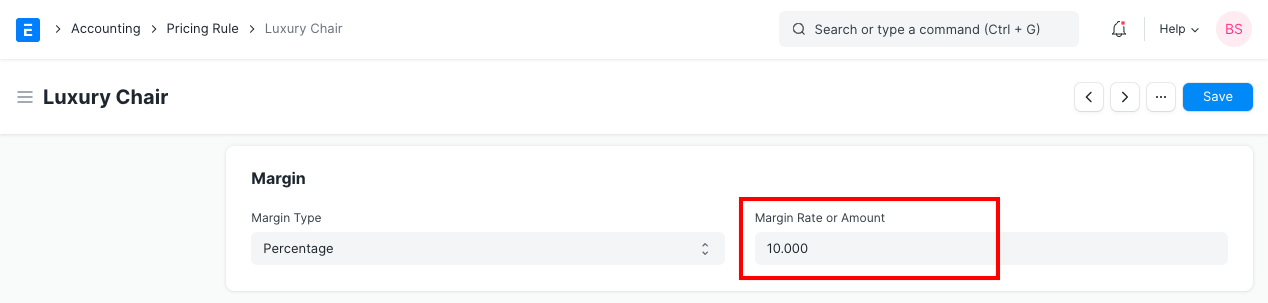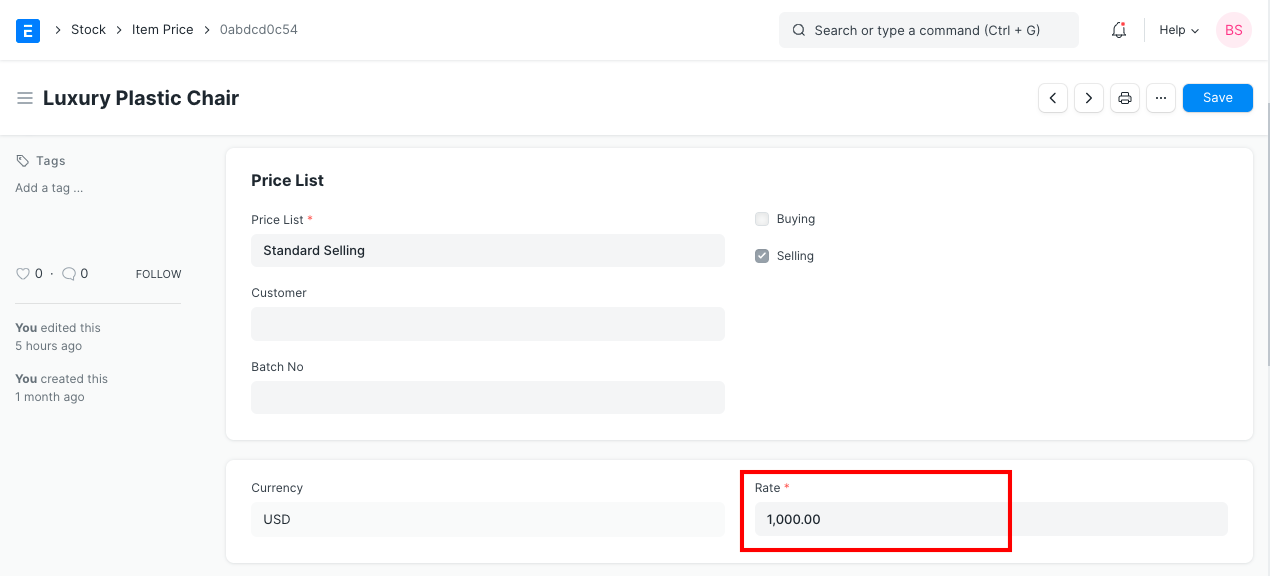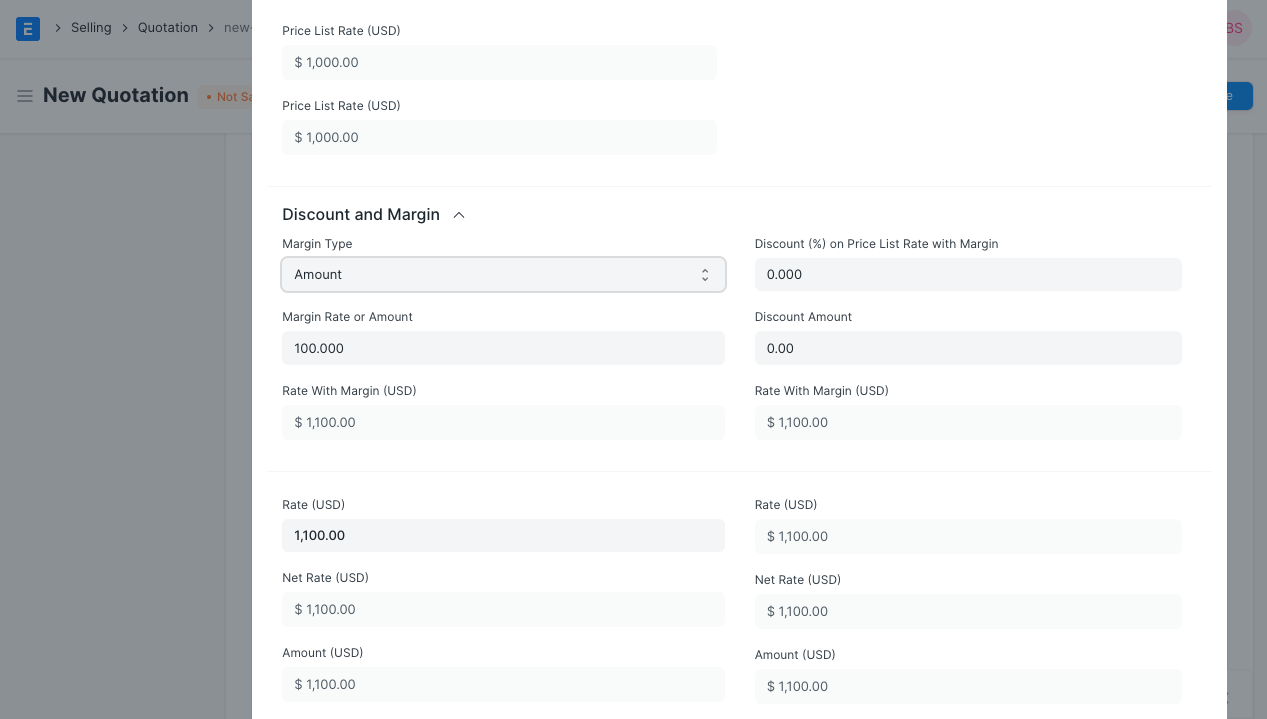User Can apply the margin on Quotation Item and Sales Order Item using following two options. 1)Price Rule: With the help of this method user can apply the margin on Quotation and Sales Order based on condition. You can find the section margin on pricing rule where a user has to select the type of margin whether it is Percentage or Amount and Rate or Amount. The system will apply the margin on quotation item and sales order item if pricing rule is enabled.

To setup Pricing Rule, go to:

`Selling > Setup > Pricing Rule` or `Accounts > Setup > Pricing Rule`

#### Adding Margin in Pricing RuleTotal Margin is calculated as follows: `Rate = Price List Rate + Margin Rate`

So, In order to apply the Margin you need to add the Price List for the Item

To add Price List, go to:

`Selling > Setup > Item Price` or `Stock > Setup > Item Price`2) Apply margin direct on Item: If user wants to apply the margin without pricing rule, they can use this option. In Quotation Item and Sales Order Item, user can select the margin type and rate or amount. The system will calculate the margin and apply it on price list rate to calculate the rate of the product.

To add margin directly on Quotation or Sales Order, go to:

`Selling > Document > Quotation`

add item and scroll down to section where you can find the Margin Type